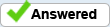# Populate a Checkbox based on Conditions

I have tried and tried and have had no success. I want to populate a checkbox based on the following script I have in a field. The Checkbox Name is NCI. The custom Calcuation in the field that gives me the result I'm looking for is as follows:

var SCLI=this.getField("SCLI_1").value
var JCLI=this.getField("JCLI_1").value
var CDI=this.getField("CDI_1").value
if ((SCLI=="N") && (JCLI=="N") && (CDI=="N")) event.value=("N");
else event.value=("Y");

I would like to Check the box named NCI if the event value = N. Is there a way to do this? Any guidance would be greatly appreciated. Thank you

Peter Stewart

Change the last few lines to:

if ((SCLI=="N") && (JCLI=="N") && (CDI=="N")) {
getField("NCI").value = "Export_Value";
event.value = "N";
} else {
event.value = "Y";
getField("NCI").value = "Off";
}

Where 'Export_Value" is the export value of the check box, which is often "Yes". This also deselects the check box if event.value does not get set to "N".

By George Johnson